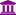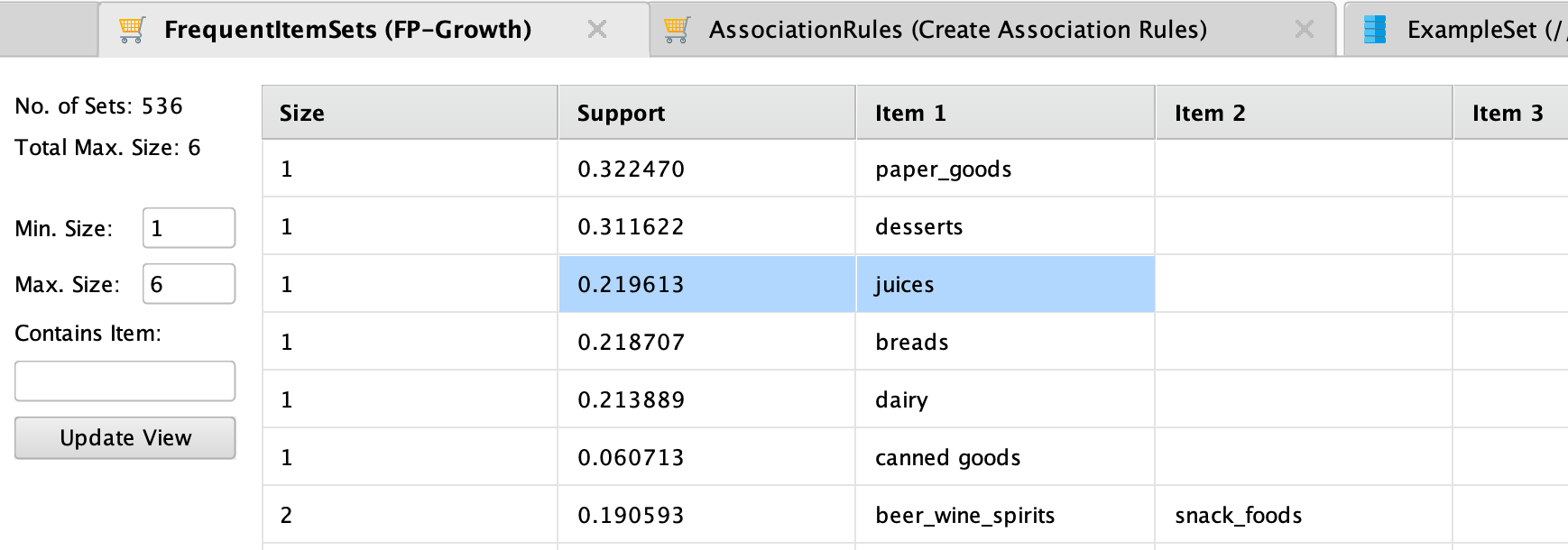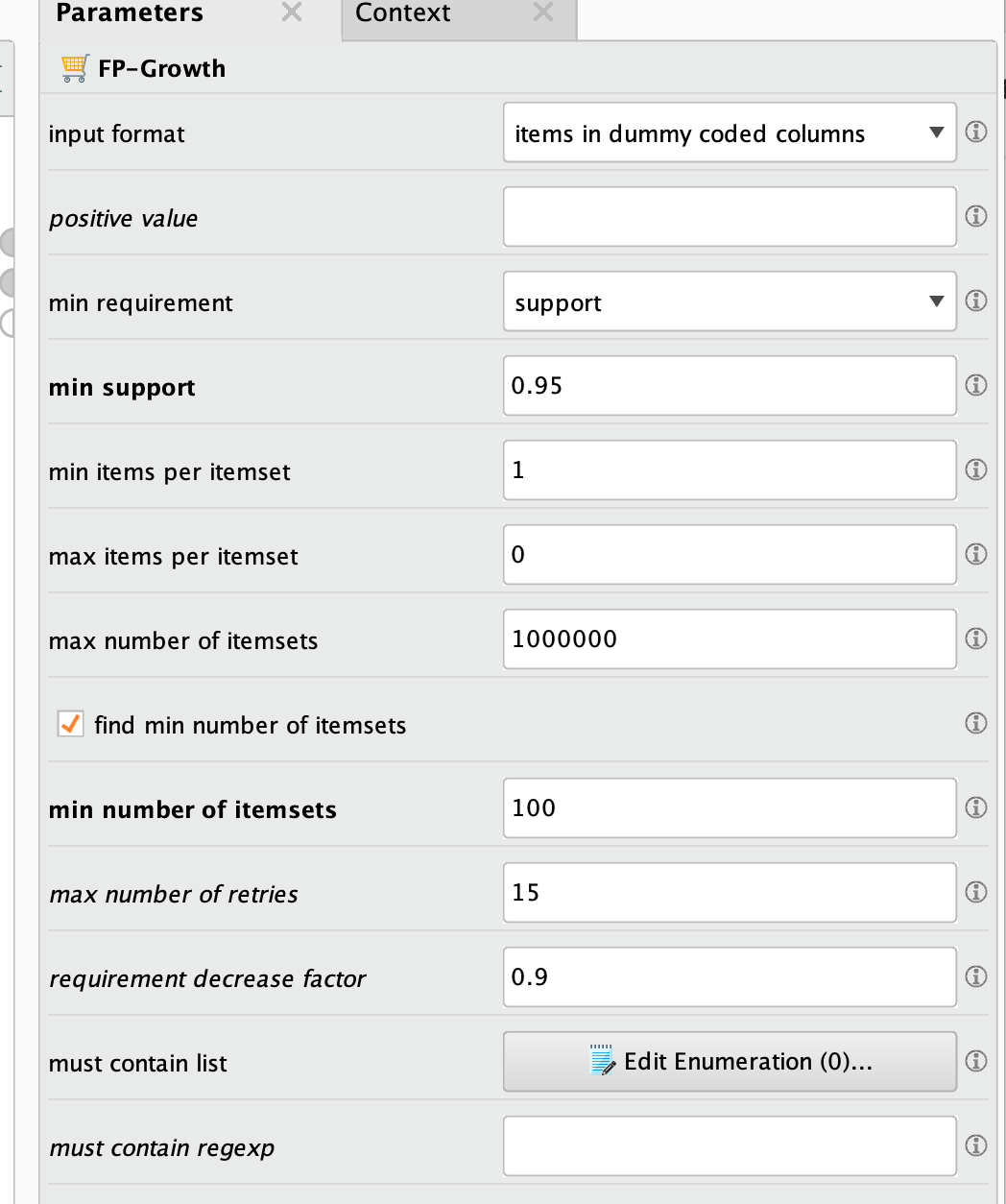# Basics of FP-Growth

Member, University Professor Posts: 60University Professor
edited March 2020 in Help
Hello all,

I am struggling quite a bit with the FP-growth operator. I got all sorts of errors (no binomial attributes when I manually set them to binomial, outputs that I cannot understand, etc). I am trying to run the smallest possible example: 2 transactions, 3 products (juice, meat and milk)! My excel file is like that:

0 0 1
0 0 1

What am I doing wrong? What are the basic errors one should avoid when using FP-Growth? I read the help page at RM on this operator and I found it extremely confusing also. Any help is appreciated, I just want to use the operator in the simples possible way.

Regards,
Bernardo

• Member, University Professor Posts: 60University Professor
Solution Accepted
Oh, now I see: this option has tow modes, and when find min number of itemsets is checked it ignores this minimum value.

Solved!!!

• Member, University Professor Posts: 60University Professor
Follow up: I have been playing with the data set of chapter 8 of the book RapidMiner: Data mining use cases and business analytics applications, which is available at http://rapidminerbook.com/
I think there is something weird going on: using the exact same steps as the author suggests, I got the same result as he did. For instance, the frequency of "juices" as a single item was 0.780, while the one for desserts was 0.312. Then I implemented the same situation, but now I used "read csv", and the "numerical to binomial" operator. The results for the frequencies were .220 for Juice, and 0.312 for desserts. I checked on Excel, using COUNT IF, and the last results seem to be the correct ones. Strange. It seems that RM is not counting those singletons properly, or some operator inverts a few of the values. I would appreciate it if someone could check that.

Best,
Bernardo
• Administrator, Employee, RapidMiner Certified Analyst, RapidMiner Certified Expert, Member Posts: 363RM Data Scientist
Hi @bernardo_pagnon,

The frequency output for "juices" is shown as 0.219613 which matches with your Excel count if results.support = (Number of times an item or itemset appears in the database) / (Number of baskets in the database)
Attached is the process for reference.

```<?xml version="1.0" encoding="UTF-8"?><process version="9.6.000">
<context>
<input/>
<output/>
<macros/>
</context>
<operator activated="true" class="process" compatibility="9.6.000" expanded="true" name="Process">
<parameter key="logverbosity" value="init"/>
<parameter key="random_seed" value="2001"/>
<parameter key="send_mail" value="never"/>
<parameter key="process_duration_for_mail" value="30"/>
<parameter key="encoding" value="SYSTEM"/>
<process expanded="true">
<operator activated="true" class="retrieve" compatibility="9.6.000" expanded="true" height="68" name="Retrieve Supermarket_Extracted" width="90" x="313" y="85">
<parameter key="repository_entry" value="//demo/FP-Growth/Supermarket_Extracted"/>
</operator>
<operator activated="true" class="set_role" compatibility="9.6.000" expanded="true" height="82" name="Set Role" width="90" x="447" y="85">
<parameter key="attribute_name" value="receipt_id"/>
<parameter key="target_role" value="id"/>
</operator>
<operator activated="true" class="numerical_to_binominal" compatibility="9.6.000" expanded="true" height="82" name="Numerical to Binominal" width="90" x="648" y="85">
<parameter key="attribute_filter_type" value="all"/>
<parameter key="attribute" value=""/>
<parameter key="attributes" value=""/>
<parameter key="use_except_expression" value="false"/>
<parameter key="value_type" value="numeric"/>
<parameter key="use_value_type_exception" value="false"/>
<parameter key="except_value_type" value="real"/>
<parameter key="block_type" value="value_series"/>
<parameter key="use_block_type_exception" value="false"/>
<parameter key="except_block_type" value="value_series_end"/>
<parameter key="invert_selection" value="false"/>
<parameter key="include_special_attributes" value="false"/>
<parameter key="min" value="0.0"/>
<parameter key="max" value="0.0"/>
</operator>
<operator activated="true" class="concurrency:fp_growth" compatibility="9.6.000" expanded="true" height="82" name="FP-Growth" origin="GENERATED_SAMPLE" width="90" x="782" y="85">
<parameter key="input_format" value="items in dummy coded columns"/>
<parameter key="item_separators" value="|"/>
<parameter key="use_quotes" value="false"/>
<parameter key="quotes_character" value="&quot;"/>
<parameter key="escape_character" value="\"/>
<parameter key="trim_item_names" value="true"/>
<parameter key="positive_value" value="true"/>
<parameter key="min_requirement" value="support"/>
<parameter key="min_support" value="0.005"/>
<parameter key="min_frequency" value="100"/>
<parameter key="min_items_per_itemset" value="1"/>
<parameter key="max_items_per_itemset" value="0"/>
<parameter key="max_number_of_itemsets" value="1000000"/>
<parameter key="find_min_number_of_itemsets" value="false"/>
<parameter key="min_number_of_itemsets" value="100"/>
<parameter key="max_number_of_retries" value="15"/>
<parameter key="requirement_decrease_factor" value="0.9"/>
<enumeration key="must_contain_list"/>
</operator>
<operator activated="true" class="create_association_rules" compatibility="9.6.000" expanded="true" height="82" name="Create Association Rules" origin="GENERATED_SAMPLE" width="90" x="916" y="34">
<parameter key="criterion" value="confidence"/>
<parameter key="min_confidence" value="0.1"/>
<parameter key="min_criterion_value" value="0.8"/>
<parameter key="gain_theta" value="2.0"/>
<parameter key="laplace_k" value="1.0"/>
</operator>
<connect from_op="Retrieve Supermarket_Extracted" from_port="output" to_op="Set Role" to_port="example set input"/>
<connect from_op="Set Role" from_port="example set output" to_op="Numerical to Binominal" to_port="example set input"/>
<connect from_op="Numerical to Binominal" from_port="example set output" to_op="FP-Growth" to_port="example set"/>
<connect from_op="FP-Growth" from_port="frequent sets" to_op="Create Association Rules" to_port="item sets"/>
<connect from_op="Create Association Rules" from_port="rules" to_port="result 1"/>
<connect from_op="Create Association Rules" from_port="item sets" to_port="result 2"/>
<portSpacing port="source_input 1" spacing="0"/>
<portSpacing port="sink_result 1" spacing="0"/>
<portSpacing port="sink_result 2" spacing="0"/>
<portSpacing port="sink_result 3" spacing="0"/>
</process>
</operator>
</process>
```

Cheers,
YY
• Member, University Professor Posts: 60University Professor
Dear YY,

thank you so much for your reply, and for taking the time to reproduce the results.
Take a look at this process. i did the same thing and the results are pretty weird.

Regards,
Bernardo

• Administrator, Employee, RapidMiner Certified Analyst, RapidMiner Certified Expert, Member Posts: 363RM Data Scientist
edited March 2020
That is because your "min support" is set way too high and there is no association rules extracted based on the threshold.You have opened duplicated threads on the same question. For easy communication and trace down the issues, please go to
https://community.rapidminer.com/discussion/45849/fp-growth-itemset-one-of-the-items-is-oversupported#latest

• Member, University Professor Posts: 60University Professor
Thank you for your reply, and sorry for opening multiple threads with the same question. I still do not get it, if the threshold is high, then the output of FP-Growth should be empty. It often happens that I put 0.95 and frequent item sets shows combinations with support 0.75, 0.6, etc. I don't see the purpose of the min support parameter if it does not help me cutting combinations below the 0.95 level.

Best,
Bernardo